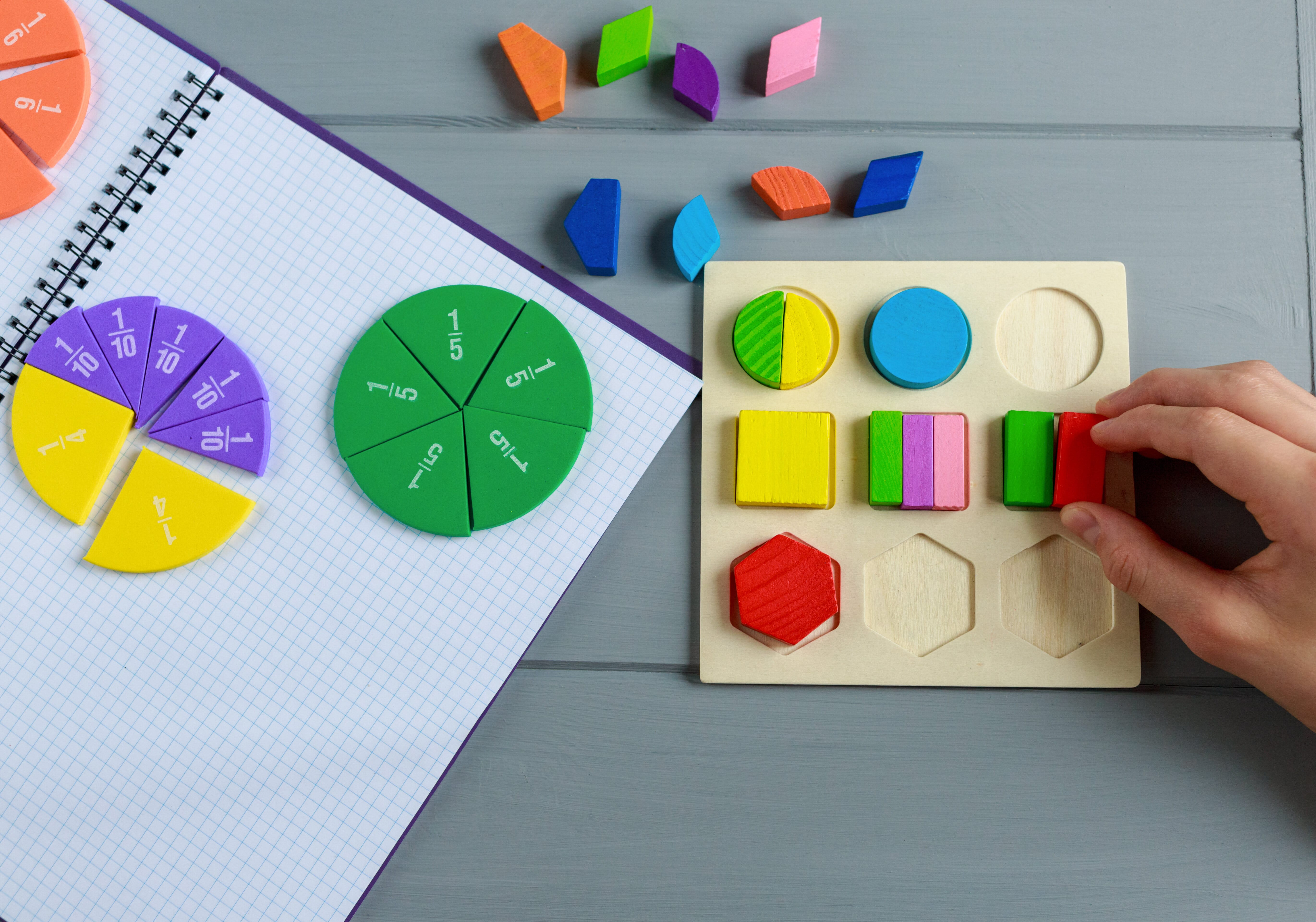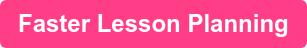# Planning the perfect fractions lesson for years 1 to 6What does a fractions lesson plan involve?

A good lesson plan teaching basic fractions lessons - or more advanced ones - in primary schools involves what all good lesson plans ought to have in common. They should include all the components of effective teaching:

• The basic objectives of the course
• The collection of teaching and learning activities is available
• How these teaching and learning activities align with assessment methods

In our guide we’ll show you some great fraction activities and worksheets, especially the much demanded multiplying fractions for year 5 and fractions lessons for years 2, 3, and 4.

Setting objectives

The objectives of your fraction lessons for primary should ideally go in parallel with the statutory requirements for KS1 and KS2 Maths in England. This document enlarges upon the milestones that students should have reached by the end of their years one through six at primary school.

Problems to solve

There is no shortage of problems to be solved when it comes to fractions. By Year 6, the Department for Education requirement says that pupils should be taught the following:

• use common factors to simplify fractions; use common multiples to express fractions in the same denomination
• compare and order fractions, including fractions greater than 1
• add and subtract fractions with different denominators and mixed numbers, using the concept of equivalent fractions
• multiply simple pairs of proper fractions, writing the answer in its simplest form [for example, 4/1 × 2/1 = 8/1 ]
• divide proper fractions by whole numbers [for example, 3/1 ÷ 2 = 6/1 ]
• associate a fraction with division and calculate decimal fraction equivalents [for example, 0.375] for a simple fraction [for example, 8/3 ]
• identify the value of each digit in numbers given to three decimal places and multiply and divide numbers by 10, 100 and 1000 giving answers up to three decimal places
• multiply one-digit numbers with up to two decimal places by whole numbers
• use written division methods in cases where the answer has up to two decimal places
•  solve problems that require answers to be rounded to specified degrees of accuracy
• recall and use equivalences between simple fractions, decimals and percentages, including in different contexts.

Relevant examples

Maths concepts that are relevant to students' own lives and can be applied as such in lesson time are highly important.

Consider that the non-statutory guidance suggests that students should be able to ‘connect their work on angles, fractions and percentages to the interpretation of pie charts’ (p. 45).

Being able to accurately add and subtract fractions for pies or sweets or anything else could probably help students win friends and influence people.

Assessments

A big high-stakes summative assessment like the SAT primary school students usually sit at the end of Key Stage Two wants to know about students’ understanding of a number of operations to do with fractions. Obviously, it comes as no surprise to say that Maths teachers ought to stick carefully to the statutory requirements and the design of the assessments. This doesn’t mean Math lessons can’t be enjoyable and fun or that they cannot use more enjoyable, quicker formative assessments to measure how well students have understood the lesson. The quick-fire Q&A round at the end of the lesson is the perfect example of this. You might want to make it more interesting, not to mention motivating for your class, by awarding small rewards for correct answers.

Why is a lesson plan important for fractions?

A fractions lesson plan separates the wheat from the chaff. It offers a clear map of effective teaching. What to teach and how to teach are the two basic questions that should be answered by your plan.

When you are clear about what topics to teach and how they should be taught, you will be able to match your teaching to the learning abilities of your students.

Annoyingly though, teaching from the front cover all the way to the end of the textbook does not meet your teaching goals but having a clear, concise lesson plan does illuminate which topics are primary as against those which are secondary.

Year 1 fractions worksheets and activities

This year group won’t typically encounter fractions yet but that doesn’t mean there are no fraction activities for year 1 you can use for planning. For example, this lesson on finding a half is a great introduction to fractions for year 1 pupils and this lesson on quarters contains lots of visualisations to help year 1 pupils grasp the basic concepts of fractions.

Year 2 fractions worksheets and activities

At year 2, a large number of schools start introducing simple fraction lessons. This year 2 fractions lesson and worksheet provides a great introduction to identifying fractions and you can discover hundreds more year 2 fractions worksheets and activities here. Games can be useful for this, too!

Year 3 fractions worksheets and activities

Some high-ability maths classes begin adding fractions in year 3. You can find more year 3 fraction review and consolidation activities here, worksheets on equivalent fractions here, or division with fractions too.

Year 4 adding and subtracting fractions worksheets and activities

For this year group, there are many offerings including building on year 3 equivalent fractions work. There’s also comparing and ordering fractions worksheets and plenty else of fractions and decimals activities for year 4 planning here.

Year 5 fractions worksheets and activities

When it comes to teaching year 5 fractions and making lesson plans, there are great lessons and worksheets available to help you introduce the concepts of adding and subtracting fractions and improper fractions and mixed numbers. When it's time to tackle fractions and decimals multiplication activities, there's a great bank of resources available that pulls resources from the likes of Max Maths, White Rose and Teacher of Primary, all into one place!

Year 6 fractions worksheets and activities

Looking to teach adding and subtracting fractions to year 6 students? Look no further than this Year 6 fractions lesson (containing both a powerpoint and worksheets) for lots of problem solving activities.

Multiplying two fractions together? There's year 6 lessons here packed full of activities and fully-planned powerpoints covering multiplying and dividing fractions.

Lesson plan templates

To speed up your lesson planning and gain access to over 30,000 resources covering the Primary curriculum, all in one place, take a look at Pango! We've partnered with dozens of award-winning publishers to bring teachers the very best content in one place! From the hundreds of lesson plans for fractions lessons to entire schemes of work, Pango's on a mission to save teachers time! Our lesson planning features allow you to save your favourite resources, create customisable lesson plans and track curriculum standards - without ever having to change tabs!